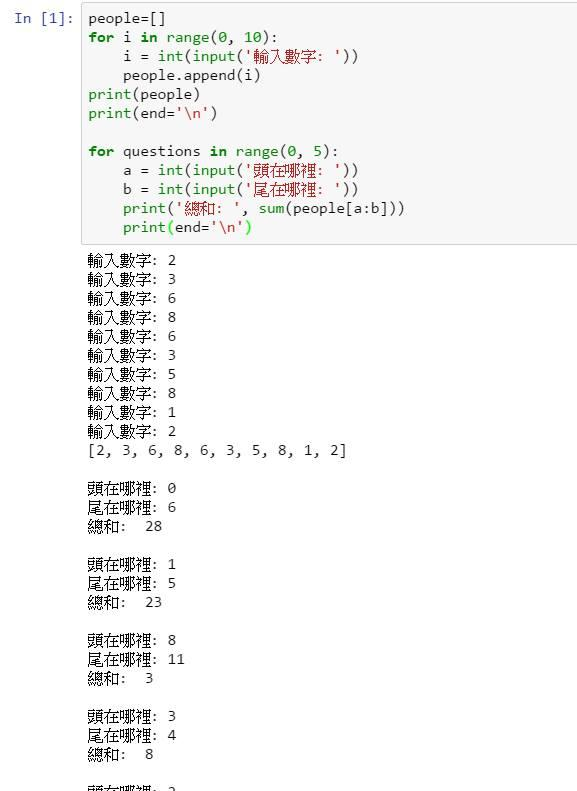## Python编程自学：一小时python入门教程

1,334次阅读

python好学吗？python入门要多久？python编程自学行吗？做为没有python编程基础的初学者肯定有各种各样的疑问！以下为Python教程学习网整理的一位台湾小姐姐写的“一小时python入门教程”，我们来看看python到底难不难，Let’s go! now!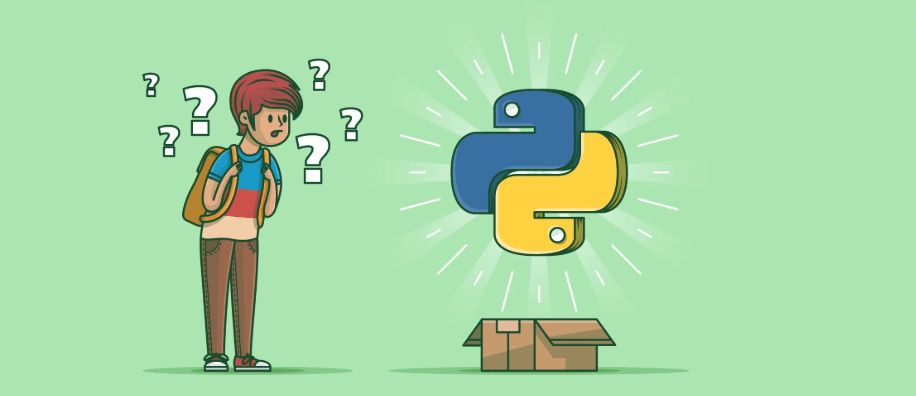Python 是一个简洁易读的语言，学习者几乎可以立刻上手，也适用于大量的商业应用上。目前已超越C/C++、Java，成为各大学课程中的主流入门程式语言，美国Top 10 Computer Science (电脑科学) 系所中便有8 所采用Python 作为入门语言。

1. 使用主机Terminal2. 下载使用Jupyter Notebook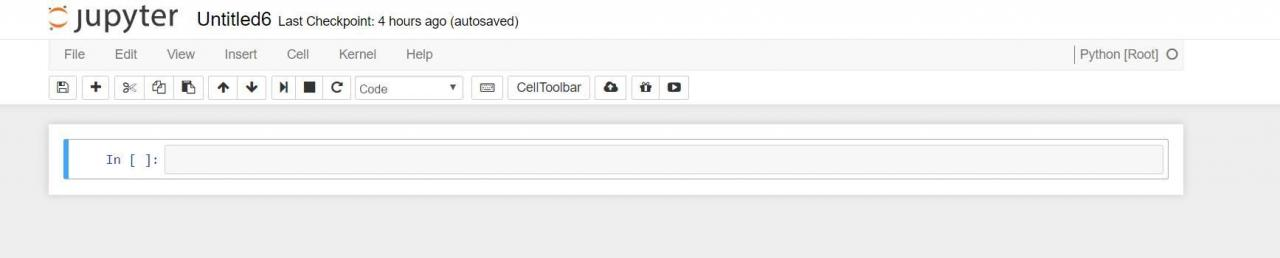1. Print：永远是跟世界打声招呼的第一个程序

print语法是在print函式中放进你要印的东西，比如print(我要印这个)。说声Hello World，跟程式的世界打声招呼吧！

`print('hello world    ')`

print的内容可以是变数(variable): print(a)，数字(init): print(1234)，或是字串(string): print(‘abc123’)。

2. Variable (变数)：一切TypeError的泉源

a = 123 , type(a)会告诉我们init (整数)。

b = ‘456’ , type(b)会告诉我们str (字串)。

c = 8.70 , type(c)会告诉我们这是一个float (浮点数)。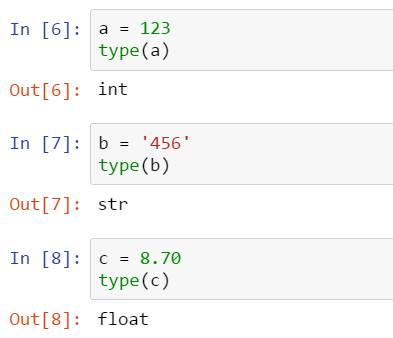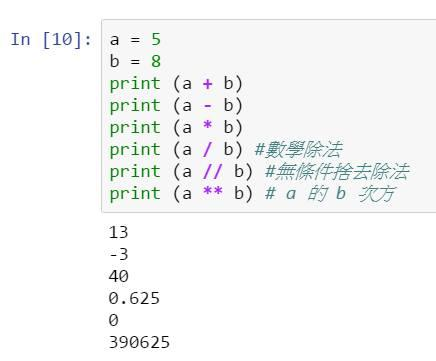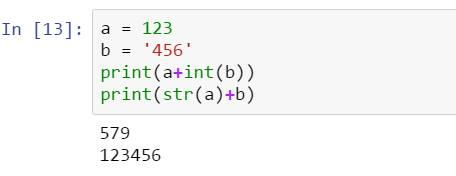3. Input：让使用者输入资料呗

input的功能是让使用者所有输入的资料会被当成字串储存起来，需要时再作转型。举例而言：

a = input() :它会等你输入，并以字串(string)的形式存入a这个变数中。● 小练习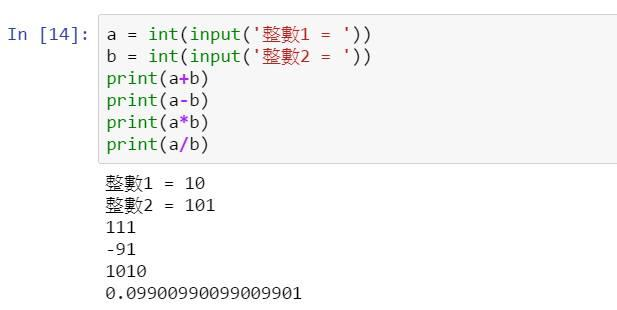4. If-Else 日常的逻辑判断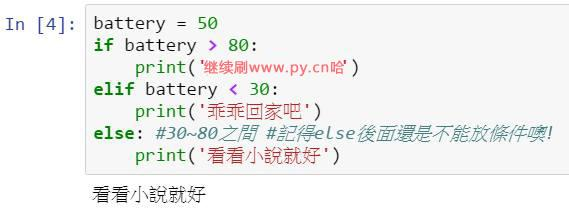if-else主要用在逻辑运算的判断上：

• > 大于
• < 小于
• >= 大于等于
• <= 小于等于
• == 等于
• != 不等于

• true 真的
• false 假的
• and 且
• or 或
• not 非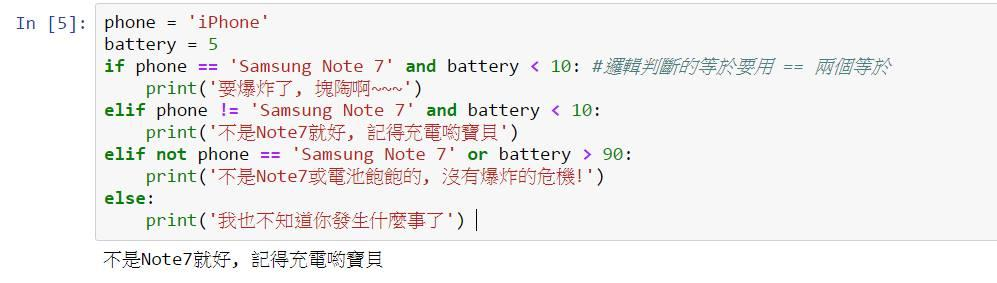● 小练习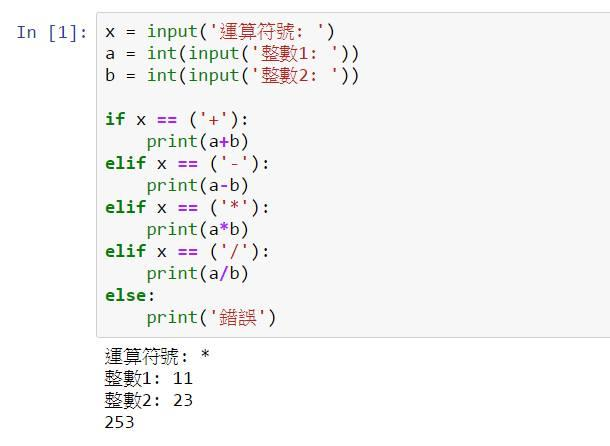5. For Loop 转圈圈吧哈姆太郎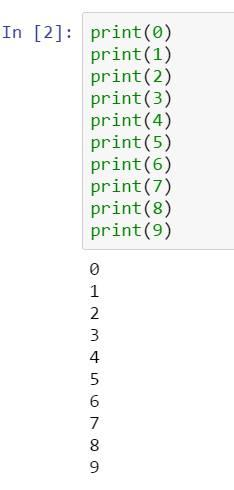for [变数名称] in range(n): (缩排) print([变数名称])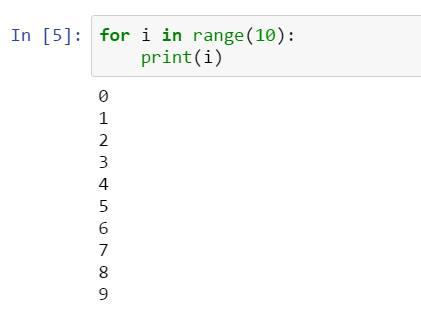```for [变量名称] in range(范围，比如从数字X到Y):
(縮排) 做一件事
(縮排) 做一件事```

● 小练习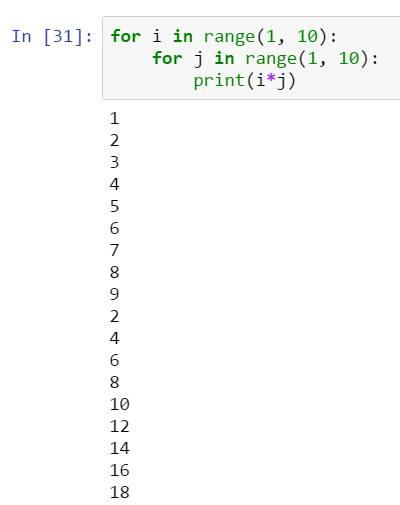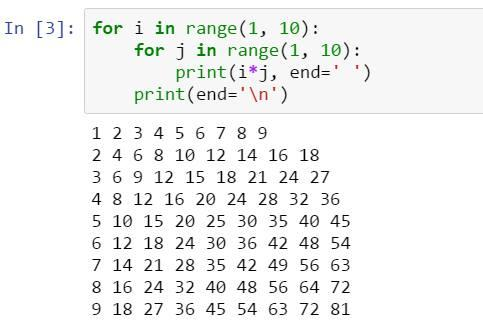range 的结构是：range(起点, 终点, 间距)，其中的间距预设为1 、比如range(0, 6) = range(0, 6, 1) 。代表从0 到5，每次加1 的意思。

(1) 从1到10、每次加2的数列;

(2) 从10到2、每次-3的数列吧！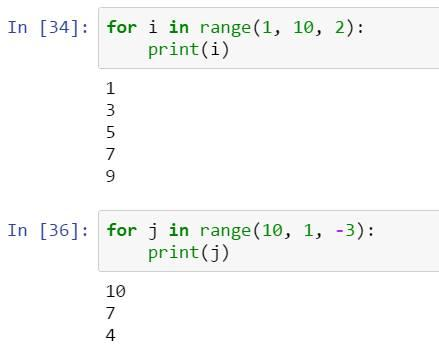● 小练习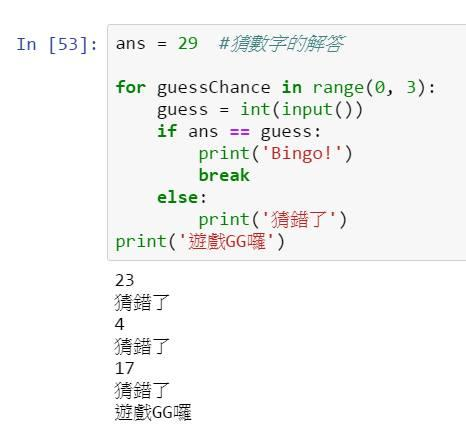6. List：能无限储存资料的格子

```a = 1
b = 2
c = 3```

… 那如果你有成千上万笔资料呢?

```in = input()
a = in
b = in```

「神说要有了List，所以有了List。」- 来自深海大凤梨里的神秘讯息。

`a = ['Lynn', 0.87, 1234, True]`

```a = [1, 3]
len(a) = 2```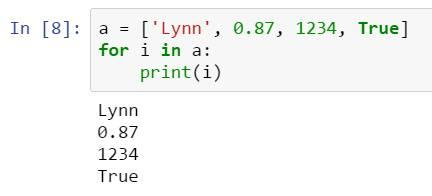● 小练习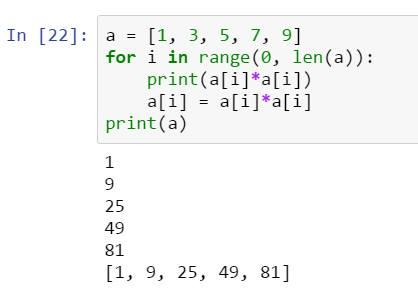• list.append(x) :把变数x塞到list的最后面
• list.insert(i, x) :把变数x塞到i这个位置上
• list.pop() :把list的最后一格丢掉
• list.pop(i) :把list的第i格丢掉
• list.remove(x) :会把第一个出现的变数x拿掉
• list.clear() :把list内的资料全部清光光

• max(list) :找出list中最大值
• min(list) :找出list中最小值
• sum(list) :找出list数字总和● 小练习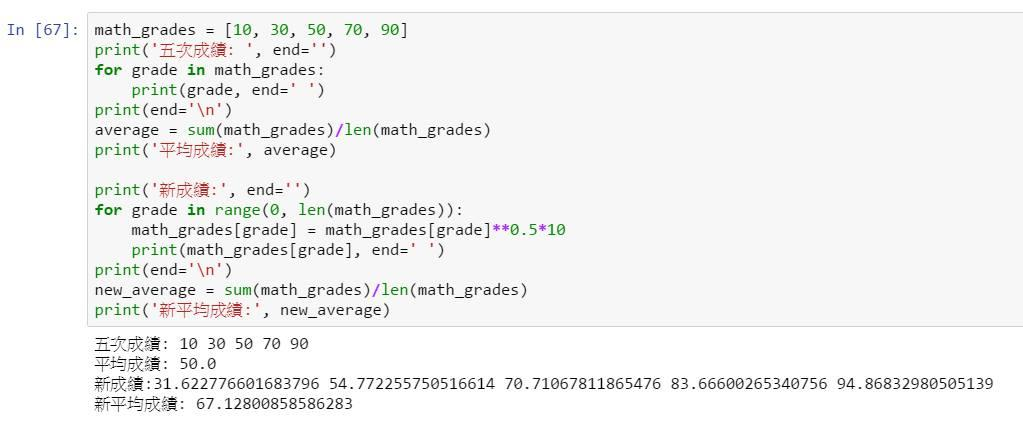Python 的slice 功能让我们能拿出List 柜子的一部份。

```list[start: end]
#拿到 list 的start ,start+1, start+2, ..., end-2, end-1```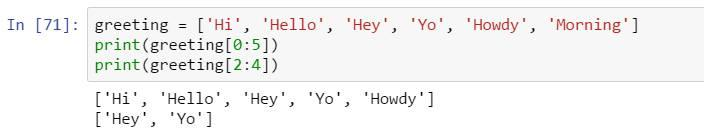• list[start: end]，start和end都可以省略不写
• start的预设为0
• end的预设为len(list)
• liest[ :end]代表0~end-1
• list[start: ]代表start~len(list)-1
• list[ : ]代表0~len(list)-1● 小练习